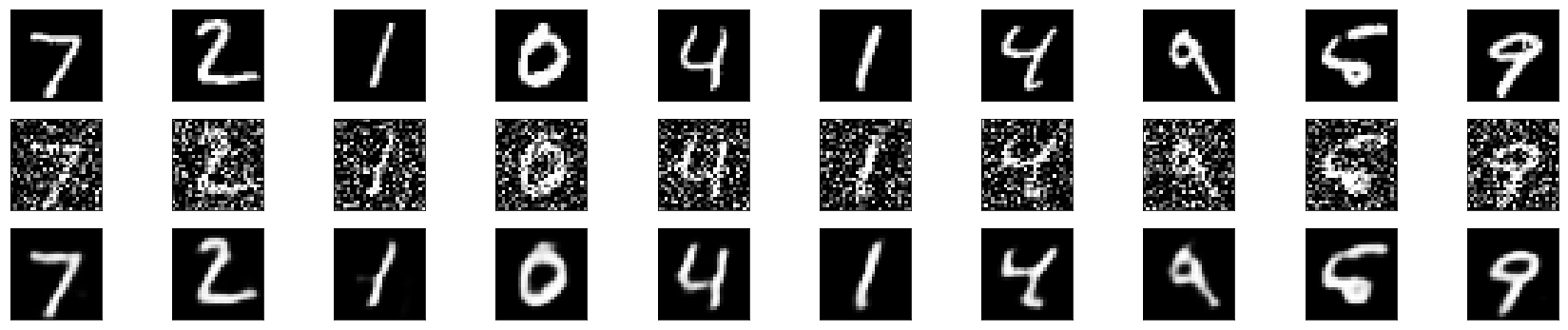# 自编码器

## 一、什么是自编码器（Autoencoder）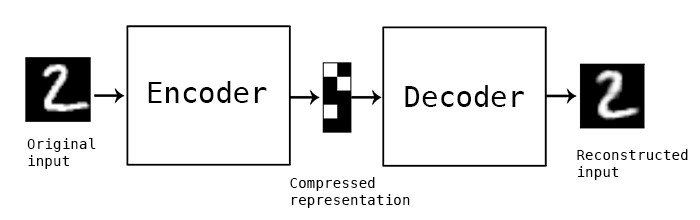1）自动编码器是数据相关的（data-specific 或 data-dependent），这意味着自动编码器只能压缩那些与训练数据类似的数据。比如，使用人脸训练出来的自动编码器在压缩别的图片，比如树木时性能很差，因为它学习到的特征是与人脸相关的。

2）自动编码器是有损的，意思是解压缩的输出与原来的输入相比是退化的，MP3，JPEG等压缩算法也是如此。这与无损压缩算法不同。

3）自动编码器是从数据样本中自动学习的，这意味着很容易对指定类的输入训练出一种特定的编码器，而不需要完成任何新工作。

## 二、几种自编码器

### 2. 正则自编码器

#### 2.3 收缩自编码器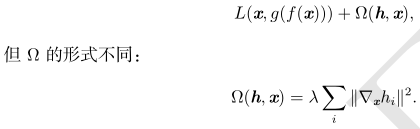### 4. 去噪自编码器

DAE 的训练准则（条件高斯p(x | h)）能让自编码器学到能估计数据分布得分的向量场 (g(f(x)) − x) ，这是 DAE 的一个重要特性。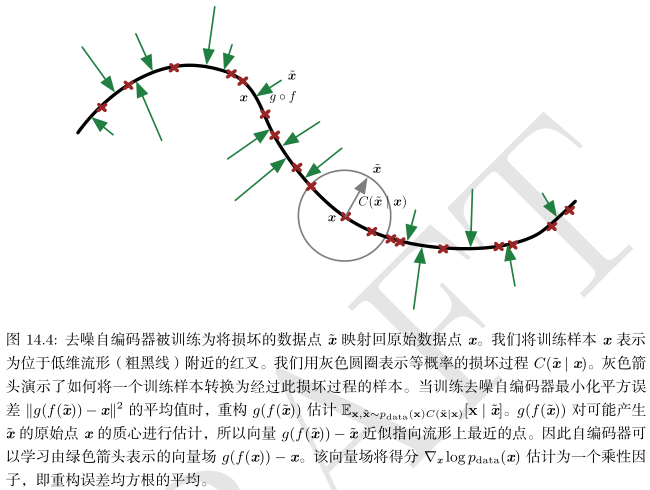### 5. 收缩自编码器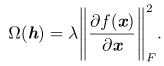## 三、使用Keras建立简单的自编码器

### 1. 单隐含层自编码器

50个epoch后，看起来我们的自编码器优化的不错了，损失val_loss: 0.1037。

from keras.layers import Input, Densefrom keras.models import Modelfrom keras.datasets import mnistimport numpy as npimport matplotlib.pyplot as plt (x_train, _), (x_test, _) = mnist.load_data() x_train = x_train.astype('float32') / 255.x_test = x_test.astype('float32') / 255.x_train = x_train.reshape((len(x_train), np.prod(x_train.shape[1:])))x_test = x_test.reshape((len(x_test), np.prod(x_test.shape[1:])))print(x_train.shape)print(x_test.shape) encoding_dim = 32input_img = Input(shape=(784,)) encoded = Dense(encoding_dim, activation='relu')(input_img)decoded = Dense(784, activation='sigmoid')(encoded) autoencoder = Model(inputs=input_img, outputs=decoded)encoder = Model(inputs=input_img, outputs=encoded) encoded_input = Input(shape=(encoding_dim,))decoder_layer = autoencoder.layers[-1] decoder = Model(inputs=encoded_input, outputs=decoder_layer(encoded_input)) autoencoder.compile(optimizer='adadelta', loss='binary_crossentropy') autoencoder.fit(x_train, x_train, epochs=50, batch_size=256,                 shuffle=True, validation_data=(x_test, x_test)) encoded_imgs = encoder.predict(x_test)decoded_imgs = decoder.predict(encoded_imgs) n = 10  # how many digits we will displayplt.figure(figsize=(20, 4))for i in range(n):    ax = plt.subplot(2, n, i + 1)    plt.imshow(x_test[i].reshape(28, 28))    plt.gray()    ax.get_xaxis().set_visible(False)    ax.get_yaxis().set_visible(False)     ax = plt.subplot(2, n, i + 1 + n)    plt.imshow(decoded_imgs[i].reshape(28, 28))    plt.gray()    ax.get_xaxis().set_visible(False)    ax.get_yaxis().set_visible(False)plt.show()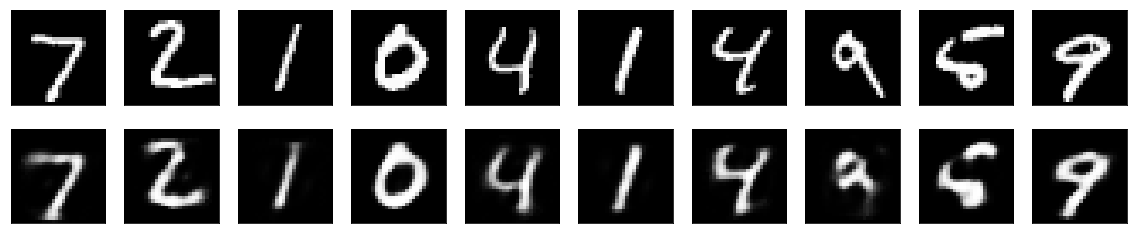### 2. 稀疏自编码器、深层自编码器

encoded = Dense(encoding_dim, activation='relu',activity_regularizer=regularizers.activity_l1(10e-5))(input_img)

input_img = Input(shape=(784,))encoded = Dense(128, activation='relu')(input_img)encoded = Dense(64, activation='relu')(encoded)decoded_input = Dense(32, activation='relu')(encoded) decoded = Dense(64, activation='relu')(decoded_input)decoded = Dense(128, activation='relu')(decoded)decoded = Dense(784, activation='sigmoid')(encoded) autoencoder = Model(inputs=input_img, outputs=decoded)encoder = Model(inputs=input_img, outputs=decoded_input) autoencoder.compile(optimizer='adadelta', loss='binary_crossentropy') autoencoder.fit(x_train, x_train, epochs=50, batch_size=256,                 shuffle=True, validation_data=(x_test, x_test)) encoded_imgs = encoder.predict(x_test)decoded_imgs = autoencoder.predict(x_test)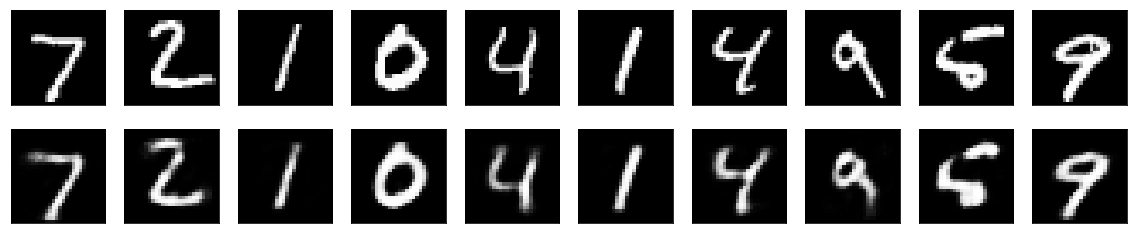### 3. 卷积自编码器：用卷积层构建自编码器

input_img = Input(shape=(28, 28, 1)) x = Convolution2D(16, (3, 3), activation='relu', padding='same')(input_img)x = MaxPooling2D((2, 2), padding='same')(x)x = Convolution2D(8, (3, 3), activation='relu', padding='same')(x)x = MaxPooling2D((2, 2), padding='same')(x)x = Convolution2D(8, (3, 3), activation='relu', padding='same')(x)encoded = MaxPooling2D((2, 2), padding='same')(x) x = Convolution2D(8, (3, 3), activation='relu', padding='same')(encoded)x = UpSampling2D((2, 2))(x)x = Convolution2D(8, (3, 3), activation='relu', padding='same')(x)x = UpSampling2D((2, 2))(x)x = Convolution2D(16, (3, 3), activation='relu')(x)x = UpSampling2D((2, 2))(x)decoded = Convolution2D(1, (3, 3), activation='sigmoid', padding='same')(x) autoencoder = Model(inputs=input_img, outputs=decoded)autoencoder.compile(optimizer='adadelta', loss='binary_crossentropy') # 打开一个终端并启动TensorBoard，终端中输入 tensorboard --logdir=/autoencoderautoencoder.fit(x_train, x_train, epochs=50, batch_size=256,                shuffle=True, validation_data=(x_test, x_test),                callbacks=[TensorBoard(log_dir='autoencoder')]) decoded_imgs = autoencoder.predict(x_test)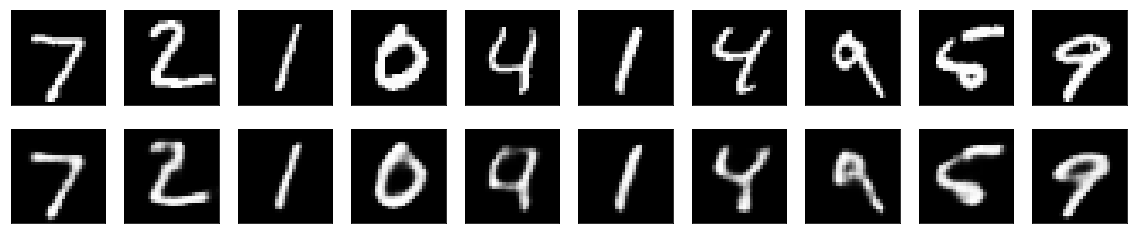### 4. 使用自动编码器进行图像去噪

from keras.layers import Input, Convolution2D, MaxPooling2D, UpSampling2Dfrom keras.models import Modelfrom keras.datasets import mnistimport numpy as npimport matplotlib.pyplot as pltfrom keras.callbacks import TensorBoard (x_train, _), (x_test, _) = mnist.load_data()x_train = x_train.astype('float32') / 255.x_test = x_test.astype('float32') / 255.x_train = np.reshape(x_train, (len(x_train), 28, 28, 1))x_test = np.reshape(x_test, (len(x_test), 28, 28, 1))noise_factor = 0.5x_train_noisy = x_train + noise_factor * np.random.normal(loc=0.0, scale=1.0, size=x_train.shape) x_test_noisy = x_test + noise_factor * np.random.normal(loc=0.0, scale=1.0, size=x_test.shape) x_train_noisy = np.clip(x_train_noisy, 0., 1.)x_test_noisy = np.clip(x_test_noisy, 0., 1.)print(x_train.shape)print(x_test.shape) input_img = Input(shape=(28, 28, 1)) x = Convolution2D(32, (3, 3), activation='relu', padding='same')(input_img)x = MaxPooling2D((2, 2), padding='same')(x)x = Convolution2D(32, (3, 3), activation='relu', padding='same')(x)encoded = MaxPooling2D((2, 2), padding='same')(x) x = Convolution2D(32, (3, 3), activation='relu', padding='same')(encoded)x = UpSampling2D((2, 2))(x)x = Convolution2D(32, (3, 3), activation='relu', padding='same')(x)x = UpSampling2D((2, 2))(x)decoded = Convolution2D(1, (3, 3), activation='sigmoid', padding='same')(x) autoencoder = Model(inputs=input_img, outputs=decoded)autoencoder.compile(optimizer='adadelta', loss='binary_crossentropy') # 打开一个终端并启动TensorBoard，终端中输入 tensorboard --logdir=/autoencoderautoencoder.fit(x_train_noisy, x_train, epochs=10, batch_size=256,                shuffle=True, validation_data=(x_test_noisy, x_test),                callbacks=[TensorBoard(log_dir='autoencoder', write_graph=False)]) decoded_imgs = autoencoder.predict(x_test_noisy) n = 10plt.figure(figsize=(30, 6))for i in range(n):    ax = plt.subplot(3, n, i + 1)    plt.imshow(x_test[i].reshape(28, 28))    plt.gray()    ax.get_xaxis().set_visible(False)    ax.get_yaxis().set_visible(False)        ax = plt.subplot(3, n, i + 1 + n)    plt.imshow(x_test_noisy[i].reshape(28, 28))    plt.gray()    ax.get_xaxis().set_visible(False)    ax.get_yaxis().set_visible(False)     ax = plt.subplot(3, n, i + 1 + 2*n)    plt.imshow(decoded_imgs[i].reshape(28, 28))    plt.gray()    ax.get_xaxis().set_visible(False)    ax.get_yaxis().set_visible(False)plt.show()